Strange Polarity Inversion in the Sun and Earth:

What could be the consequences on the Earth rotation?

Report written by H. Sayoud

Introduction

Recently, scientists revealed that the sun and earth have reversed their magnetic polarities (i.e. North becomes south and vice-versa). Another strange fact is that all the solar planets and even the sun itself spin in the opposite direction of the clock (i.e. counter-clockwise), except Venus, which has an abnormal rotation (reversed rotation) leading to see the sun rising from the west. Some reflections argue that the same phenomenon (reverse rotation) could happen to the earth leading to see the sun rising from the west one day. This investigation examines the possibilities of such an inversion and the scientific analysis of the prediction of the Prophet on the matter.

I. Case of the Sun

It seems that the sun’s magnetic field changes polarity approximately every 11 years, according to NASA resources (ref: www.nasa.gov –Article: The Sun's Magnetic Field is about to Flip, 2013- and https://www.youtube.com/watch?v=B4UtVo7-yJA ). See the two following figures.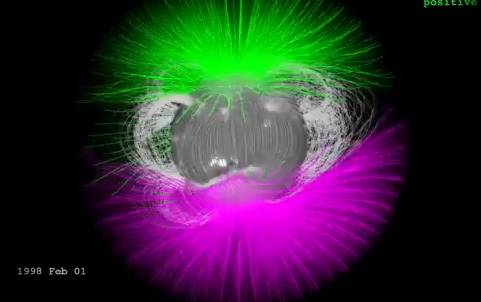Figure 1: Photo of the Sun taken in 1998, showing a positive pole (South) in green at the top of the sun and a negative pole (North) in magenta at the bottom. NASA courtesy.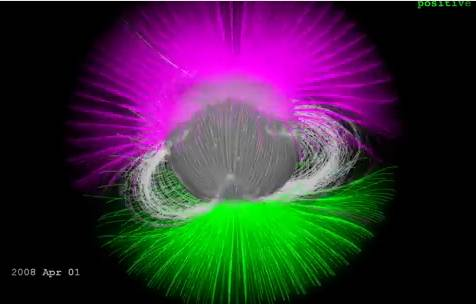Figure 2: Photo of the Sun taken in 2008 (i.e. 10 years later), showing a negative pole (North) in magenta at the top of the sun and a positive pole (South) in green at the bottom. NASA courtesy.

II. Case of the Earth

According to their article published in the International Geophysical Journal (2014), the authors: Leonardo Sagnotti (Italy), Giancarlo Scardia (Italy), Giancarlo Scardia (Italy & Brasil), Joseph C. Liddicoat (USA), Sebastien Nomade (France), Paul R. Renne (USA) and Courtney J. Sprain (USA), reported that there was an extremely rapid direction change during a polarity reversal of the earth, which occurred several thousands of years in the past (Reference: International Geophysical Journal 2014/199, pages: 1110-1124, Oxford University Press. Title: Extremely rapid directional change… polarity reversal). In other words, they show that the North Pole was in the South location and the South one in the North location. See figures 3 and 4.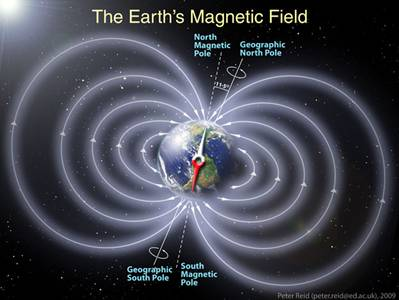Figure 3: Photo showing the migration of the north magnetic pole towards the south. It is continuously moving away from the actual north geographical pole. NASA courtesy & Peter Reid copyright.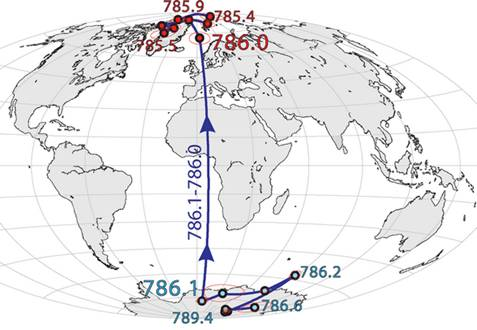Figure 4: Results of the scientific article reported by Leonardo Sagnotti et al. showing that the position of the north magnetic pole was shifted from the south to the north in the past.

III. What could be the consequences on the Earth rotation direction?

We are observing three strange aspects about the earth rotation:

•  It has been shown that the rotation speed of the earth is continuously decreasing (reference: Why is Earth rotating? by Dr Marc, www.nasa.gov);
• Furthermore, the researchers expect a polarity reversal of the earth in the future (reference: see section 2 above);
• Moreover, it has been strangely noticed that the rotation of Venus is opposite to most of the solar system movements. Some researchers claim that its previous rotation was regular such as most of the planets, but Venus had probably reversed its direction due to some physical phenomena (reference: Why Venus Spins the Wrong Way?  by Harald Franzen, www.scientificamerican.com).

All these observed aspects suggest that it could come a day when the Earth will also change the direction of its rotation, or at least, we can state that this phenomenon is quite likely to occur, as it might be occurred for Venus before.

IV. Possible scenarios

a- Deceleration of the earth rotation: the earth rotation speed is continuously decreasing, hence a continuous deceleration should lead to a zero speed one day!

However, from a mechanics point of view, the speed could not stop abruptly ant the acceleration could not change roughly either. That is, a continuity in speed and acceleration is expected.

For instance if the speed follows the following mathematical function: S(t)=S0.Cos(2pi.F0.t), which is very common in mechanical movements, the rotation speed of the earth is S0 at the time t=0 and which is maximal, leading for example to a period of about 24 hours/day at that initial time.

After a time t1 equal to the quarter of the rotation acceleration period, namely ¼.1/F0, the new speed becomes:

S(t)=S0.Cos(2pi.F0.1/4.1/F0) =S0.Cos(pi/2) =0.

In other words, at t=t1 (the quarter of the acceleration period), the rotation of the earth should stop.

However, due to the inertia continuity in the Universe, the movement should continue again according to the previous equation, so thereafter, after a very short time epsilon (epsilon <<1) corresponding to a time t2=t1+epsilon, the new speed should become:

S(t2)= S0.Cos(2pi.F0. (1/4.1/F0 + epsilon) ) = S0.Cos(pi/2 + epsilon’).

where epsilon’ =  2pi.F0.epsilon and is also very small (epsilon’ << 1).

Now, we remark that the estimated speed S.Cos(pi/2 + epsilon’) is obviously negative. For instance if epsilon’ = 0.1, then the value of the speed is –0.099S0 or approximately -0.1S0.

Consequently, the new speed at t2=t1+epsilon is negative. That means that the earth rotation will be reversed at that time and should rotate quite slowly in a clockwise direction such as Venus. In this case the sun will begin to rise in the west. See the following figure for concreteness.

Figure 5: Earth rotation inversion (simulation). Special thanks to bestanimations.com.

b- Flip of the earth axis: the earth rotation axis is tilted and may continue to deviate from the perfect vertical plane in the future. Maybe, it could continue its tilt until the complete reversion of the planet by an angle of about 180 degrees.

If such a scenario came to occur, then the rotation of the earth would be clockwise such as Uranus in the first time and Venus at a final stage.

However, in this case, the sun will still seem to rise from the east. See the following figure for concreteness.

Figure 6: Earth flip (simulation).

V. What is the opinion of the Prophet on this point?

Interestingly, the rise of the sun from the west is mentioned in the Hadith by the Prophet. In fact, the Prophet Mohamed (PBUH) said: "One of the signs of the hour (the judgment day) is the fact that the sun will rise from the west” (reference: Hadith, Sahih Muslim). In other words, the rise of the sun from the west is probably due to the reversed rotation of the Earth, as it is the case on Venus. See figure 7.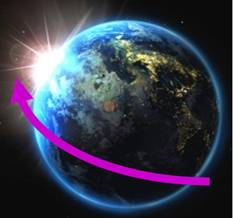Figure 7: A reverse rotation of the earth leads to see the sun rising from the west.

VI. Discussion

We are facing extremely complex astronomical facts that are very hard to demonstrate, however, day after day, the scientific discoveries on several astronomical and geological aspects make the reflection further and further in favor of the prediction of the Prophet (i.e. on the possible reversion of the earth rotation). I do not argue that these scientific results prove that prediction, but (evidently) they make it quite probable or, at least, scientifically possible.

References

 www.nasa.gov –Article: The Sun's Magnetic Field is about to Flip, 2013-